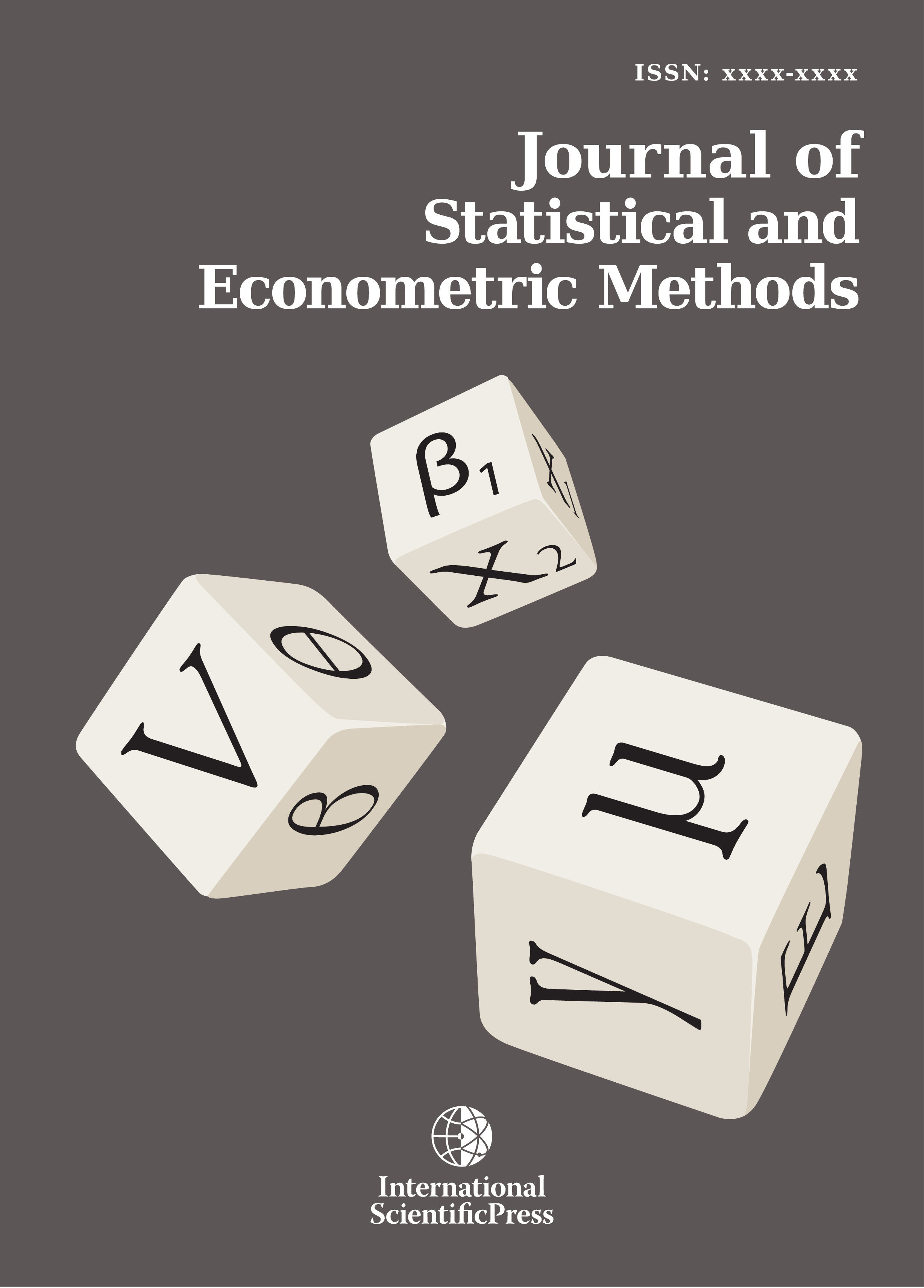# Journal of Statistical and Econometric Methods

#### Assessment on some estimators of a system of simultaneous equation model on the influence of measurement errors in variables of the model

•[ Download ]
• Abstract

This paper discusses a Monte Carlo experiment carried out to assess and examine the performance on the finite sample properties of ordinary least squares, indirect least squares, two stage least squares, and three stage least squares estimates of the parameters of the simultaneous equation model on the influence of measurement errors. A system of three just identified equations is set up and application of the four least square techniques is carried out at four categories; when the variables in the models are free from errors, when only the exogenous variables in the model are contaminated with errors, when only the endogenous variables in the model are contaminated with errors, and when both variables are contaminated. From the analysis, it was observed that the estimates of the parameters in the model at each technique vary at different models. It was also observed that 2SLS is the best estimator when all variables in the model are free from errors. 3SLS took the advantage and became best when only the exogenous variables are contaminated. ILS is best when only the endogenous variables are contaminated. And when both variables are contaminated, ILS and 2SLS became similar and best.

Mathematics Subject classification: 62J99

Keywords: Simultaneous equation, exactly identified equation, Monte-Carlo, Measurement Errors, estimators, endogenous and exogenous variablesISSN: 2241-0376 (Online)
2241-0384 (Print)